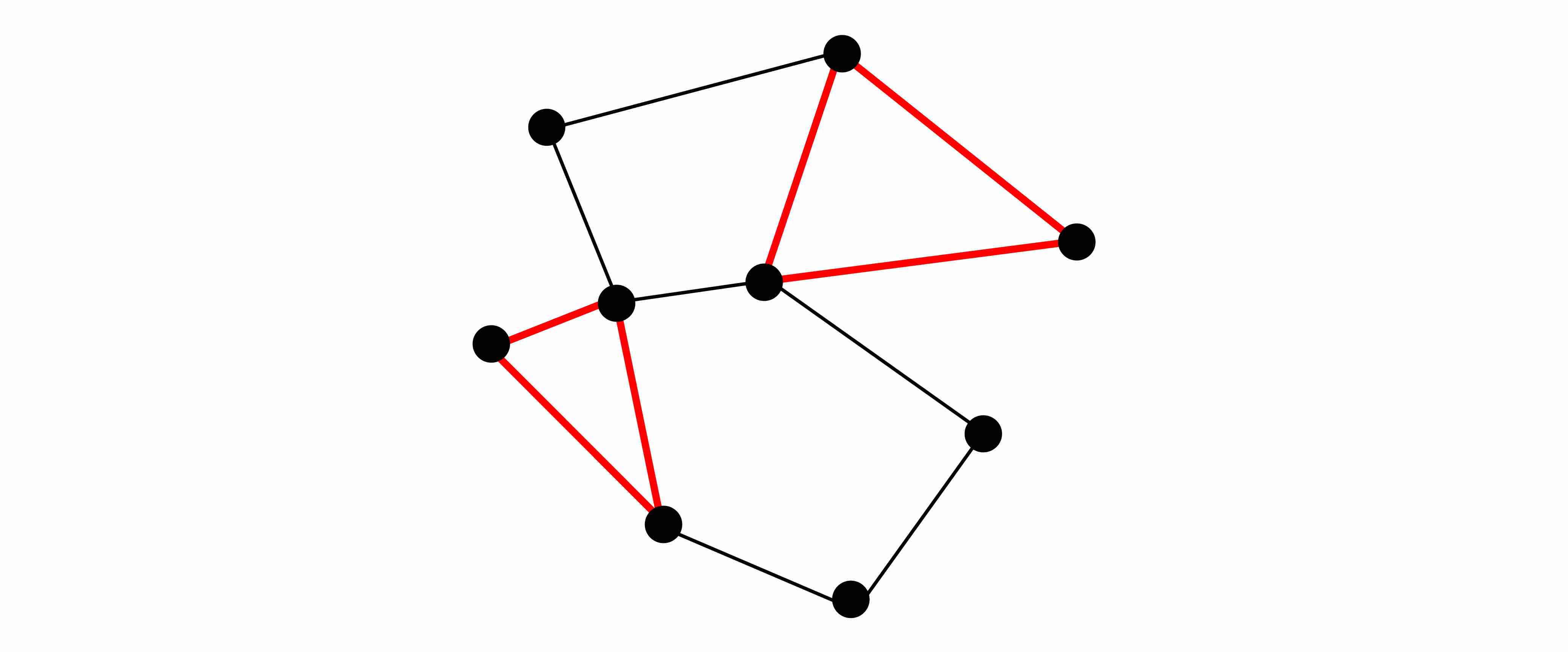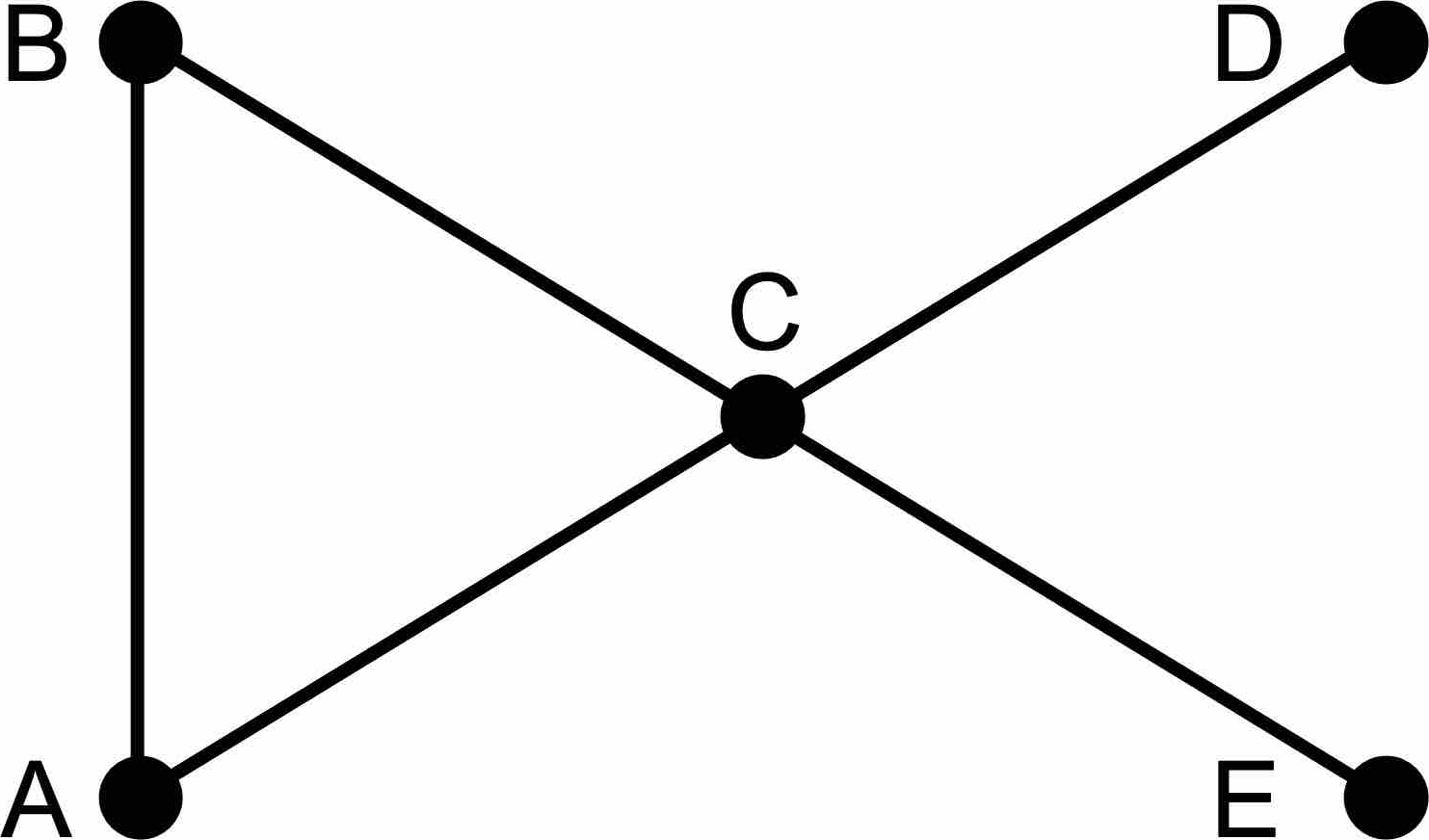## Motivation

One day at work with Humanyze, I came across a particularly elegant and mysterious method to count the number of triangles in a node graph (in python).

Suppose `S` is a numpy array of the square matrix representation of an undirected node graph (with no self edges), then

``````triangles_count = S.dot(S).dot(S).trace() / 6
``````

This is saying “the number of triangles in `S` is equal to first calculating `S``S``S` via matrix multiplication, and then getting the trace of the result (i.e., the sum of the values on diagonal) and dividing it by 6.”

But what does any of that have to do with triangles? I mean, the trace, really?

[Update: if you’re curious about counting more than just triangles, also check out my next post on counting triangles, quadrilaterals, and pentagons]

## Why it works

Let’s consider a simple node graph### Starting with S

The matrix representation `S` of this node graph would be the following

``````S =

A   B   C   D   E
A   0   1   1   0   0
B   1   0   1   0   0
C   1   1   0   1   1
D   0   0   1   0   0
E   0   0   1   0   0
``````

This is where it’d like to make an important insight that will be more useful later: each `(i, j)` represents the number of ways you can cross exactly 1 edge when you start at `i` and end at `j`.

For example, there’s no way start at `A` and end at `A` while crossing one edge, so that’s why we see 0 in the `(A, A)` position. But there’s just one way you can get from `A` to `B` via one edge, so that’s why we see a 1 in the `(A, B)` position. Notice also that there’s no way to cross just one edge and get from `A` to `E`, so that’s why there’s a 0 in the `(A, E)` position.

### S • S or S2

Let’s review how each element of S2 would be calculated.

`(A, A)` of S2 is derived from the following

``````# first row                    # first column

A   B   C   D   E              A
A   0   1   1   0   0          A   0
B   1
(dot)  C   1
D   0
E   0

AA * AA = 0 * 0 = 0
AB * BA = 1 * 1 = 1
AC * CA = 1 * 1 = 1
AD * DA = 0 * 0 = 0
+   AE * EA = 0 * 0 = 0
-----------------------
2
``````

Notice what this means in terms of our graph. The first row represents everywhere `A` leads to. The first column represents all the nodes that lead to `A`. Thus, this calculation counts the number of ways you can leave `A` traveling along one edge and then traveling another edge (perhaps the same one) and return to `A`. Namely, there are 2 ways: `A` -> `B` -> `A` and `A` -> `C` -> `A`.

Now let’s derive `(A, B)` of S2

``````# first row                    # second column

A   B   C   D   E              B
A   0   1   1   0   0          A   1
B   0
(dot)  C   1
D   0
E   0

AA * AB = 0 * 1 = 0
AB * BB = 1 * 0 = 0
AC * CB = 1 * 1 = 1
AD * DB = 0 * 0 = 0
+   AE * EB = 0 * 0 = 0
-----------------------
1
``````

Again, the first row represents everywhere `A` leads to, but the second column represents all the nodes that lead to `B`. Thus, this calculation counts the number of ways you can leave `A` traveling along one edge and then traveling another edge (perhaps the same one) and end up at `B`. Namely, there is only 1 way: `A` -> `C` -> `B`.

Completing the product results in the following

``````S.dot(S) =

A   B   C   D   E
A   2   1   1   1   1
B   1   2   1   1   1
C   1   1   4   0   0
D   1   1   0   1   1
E   1   1   0   1   1
``````

For the reasons described, S2 has the following characteristics: each `(i, j)` represents the number of ways you can cross exactly 2 edges when you start at `i` and end at `j`.

### S • S • S or S3

``````S.dot(S).dot(S) =

A   B   C   D   E
A   2   3   5   1   1
B   3   2   5   1   1
C   5   5   2   4   4
D   1   1   4   0   0
E   1   1   4   0   0
``````

Following the same reasoning as with S2, our S3 has the following characteristics: each `(i, j)` represents the number of ways you can cross exactly 3 edges when you start at `i` and end at `j`.

Now it’s time for the fun part.

### Counting Triangles

We see that the 2 in the `(A, A)` position of S3 tells us that there are two ways to start at `A`, cross three edges, and then end up back at `A`. Wouldn’t you know, this is saying that there are two ways to walk in a triangle starting and ending at `A`. In fact, those two ways represent the same triangle but simply traversed in different directions: `A` -> `B` -> `C` -> `A` and `A` -> `C` -> `B` -> `A`.

Notice also that the 2 in the `(B, B)` position would also represent the same triangle but simply starting/ending at `B`. Likewise for the 2 in the `(C, C)` position.

With this in mind, every triangle in our node graph will be counted 6 times as we walk along the diagonal. No other paths represent triangles except those on the diagonal.

Therefore, `S.dot(S).dot(S).trace() / 6` represents the number of triangles!

## Code Examples

Below I have embedded the html version of a Jupyter notebook that demonstrates the example we’ve worked through here, as well as one additional example.

Here is a link to a full-page view of the notebook. Or you can download a copy of the .ipynb file here.